# GRAPHING MULTIPLE FORMS OF QUADRATIC FUNCTIONS UNIT 3

• Slides: 39GRAPHING MULTIPLE FORMS OF QUADRATIC FUNCTIONS UNIT 3 DAY 2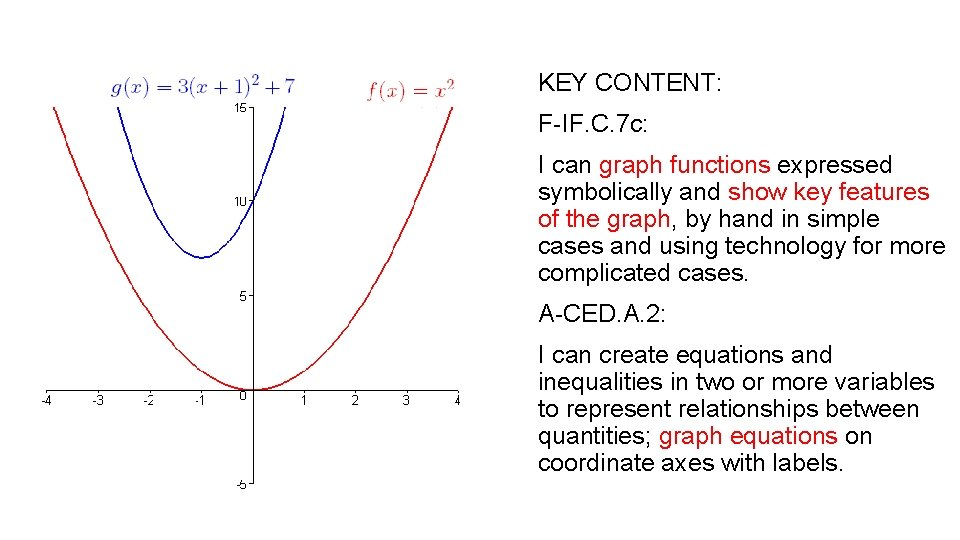KEY CONTENT: F-IF. C. 7 c: I can graph functions expressed symbolically and show key features of the graph, by hand in simple cases and using technology for more complicated cases. A-CED. A. 2: I can create equations and inequalities in two or more variables to represent relationships between quantities; graph equations on coordinate axes with labels.Today I will review how to graph quadratic equations in different forms.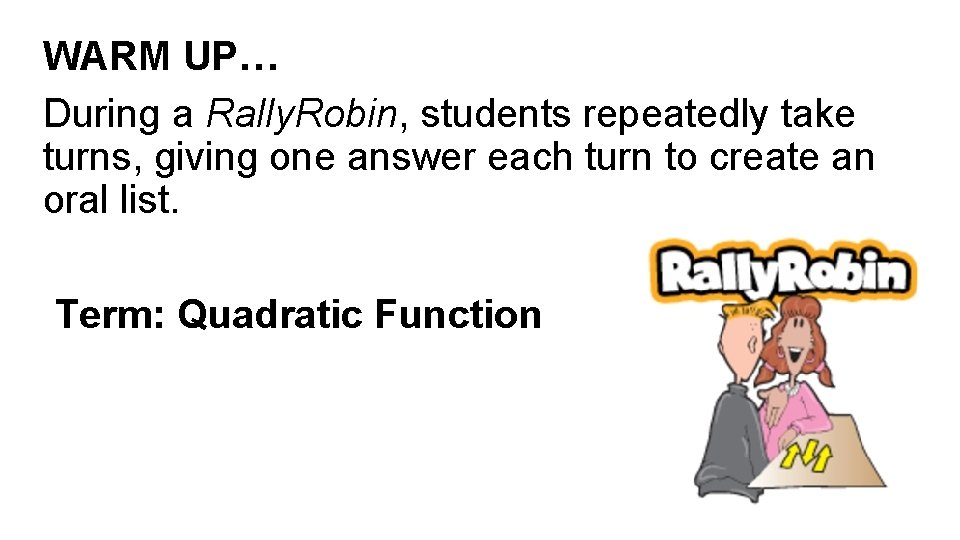WARM UP… During a Rally. Robin, students repeatedly take turns, giving one answer each turn to create an oral list. Term: Quadratic FunctionThe graph of a quadratic function is a symmetrical U shape called a parabola. Using the discriminant of the quadratic formula, , you can determine if the quadratic function has… Two real roots – if the discriminate is positive One real root – if the discriminate is equal to zero Two complex roots – if the discriminate is negative The graph of a quadraticWARM UP… During a Rally. Robin, students repeatedly take turns, giving one answer each turn to create an oral list Term: Parabola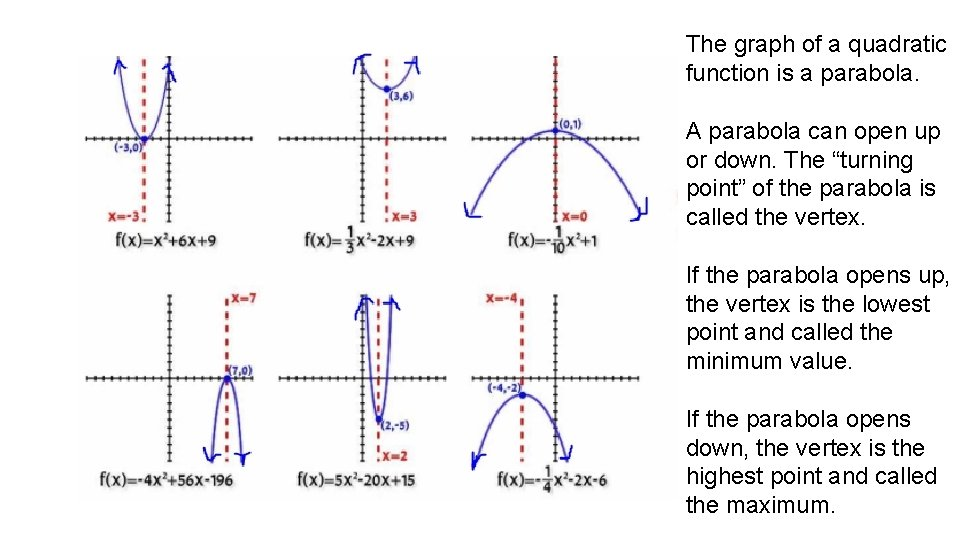The graph of a quadratic function is a parabola. A parabola can open up or down. The “turning point” of the parabola is called the vertex. If the parabola opens up, the vertex is the lowest point and called the minimum value. If the parabola opens down, the vertex is the highest point and called the maximum.After allowing students to write down their meaning of the terms, watch a portion of the video below (0: 00 -1: 57) http: //study. com/academy/lesson/what-is-a-quadratic-equationdealfinition-examples. html A quadratic function is one of the form f(x) = ax 2 + bx + c, where a, b, and c are numbers with a not equal to zero. The graph of a quadratic function is a curve called a parabola. In quadratic functions, parabolas may only open upward or downward and vary in "width" or "steepness", but they all have the same basic "U" shape. Optional SlideWARM UP… During a Rally. Robin, students repeatedly take turns, giving one answer each turn to create an oral list Term: VertexThe vertex of a parabola is the point where the parabola crosses its line of symmetry. The vertex is the highest or lowest point on the graph depending on where the parabola opens up or down. For a given quadratic y = ax 2 + bx + c, the vertex (h, k) is found by computing h = – b/2 a, and then evaluating y at h to find k.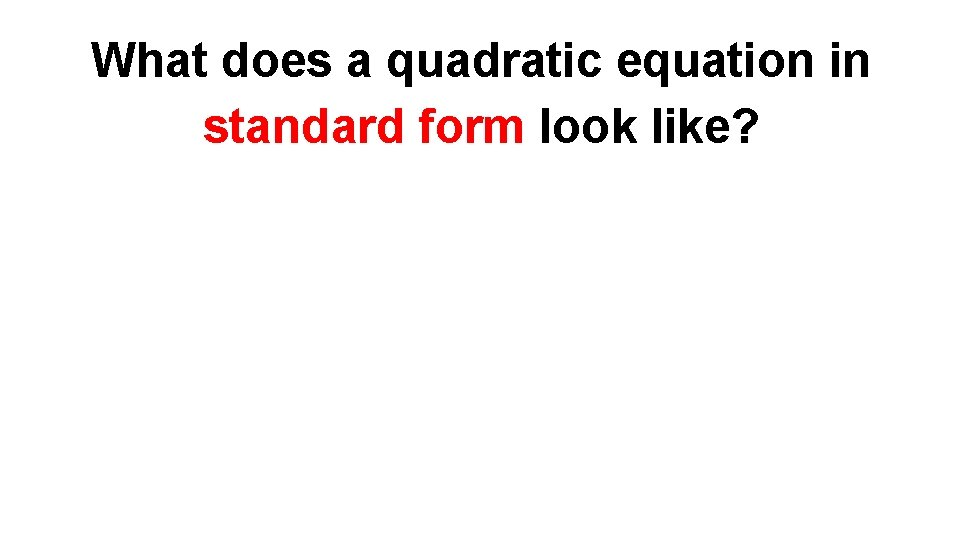What does a quadratic equation in standard form look like?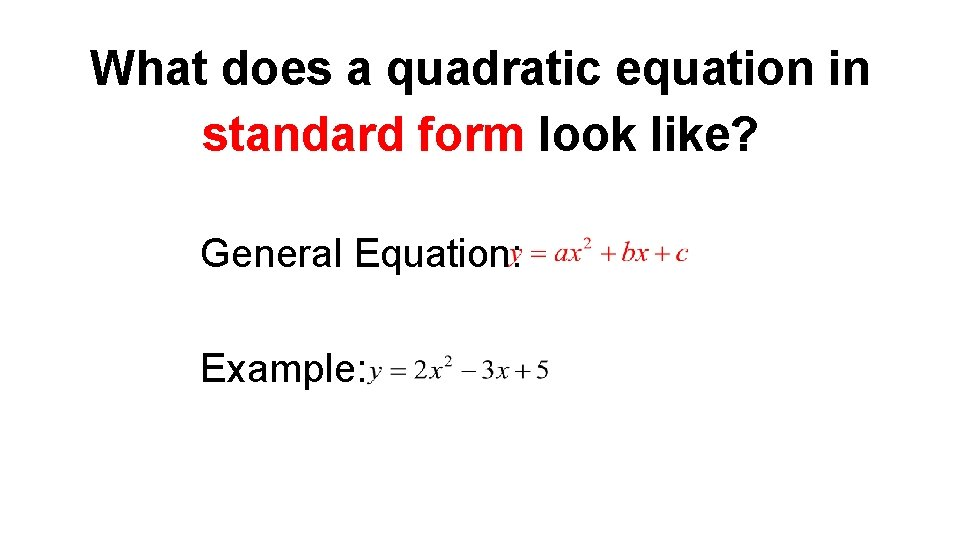What does a quadratic equation in standard form look like? General Equation: Example: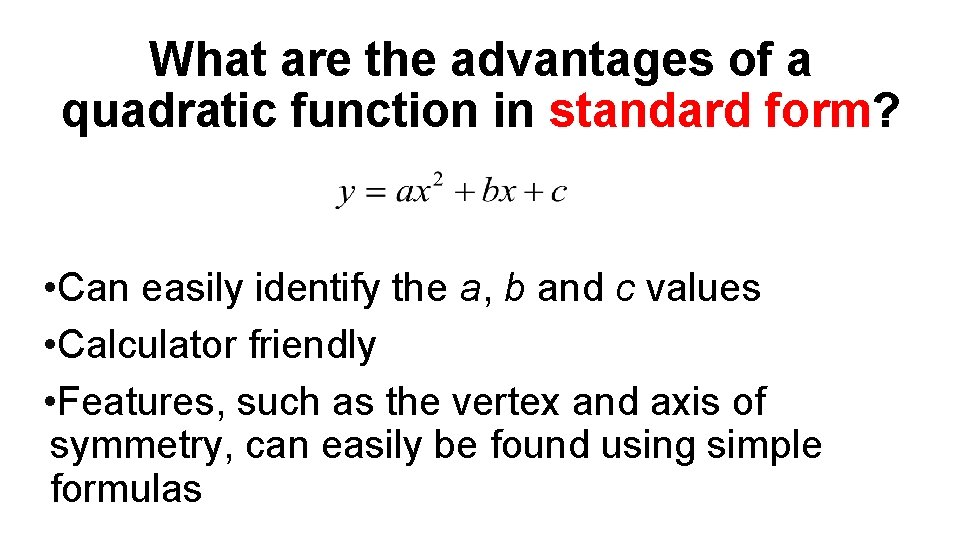What are the advantages of a quadratic function in standard form? • Can easily identify the a, b and c values • Calculator friendly • Features, such as the vertex and axis of symmetry, can easily be found using simple formulas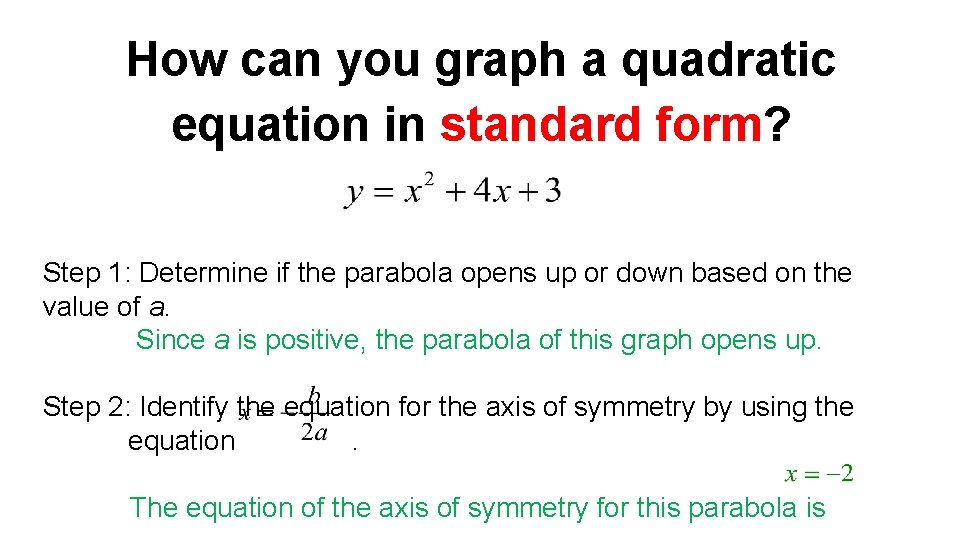How can you graph a quadratic equation in standard form? Step 1: Determine if the parabola opens up or down based on the value of a. Since a is positive, the parabola of this graph opens up. Step 2: Identify the equation for the axis of symmetry by using the equation. The equation of the axis of symmetry for this parabola isHow can you graph a quadratic equation in standard form? The axis of symmetry divides a parabola in half. The vertex always occurs along the axis of symmetry. For a parabola that opens up, the vertex occurs at the minimum point or the lowest point on the graph. Step 3: Calculate the y-coordinate of the vertex, by plugging in x value into the original equation and solving for y. Then graph the vertex.How can you graph a quadratic equation in standard form? axis of symmetry: vertex: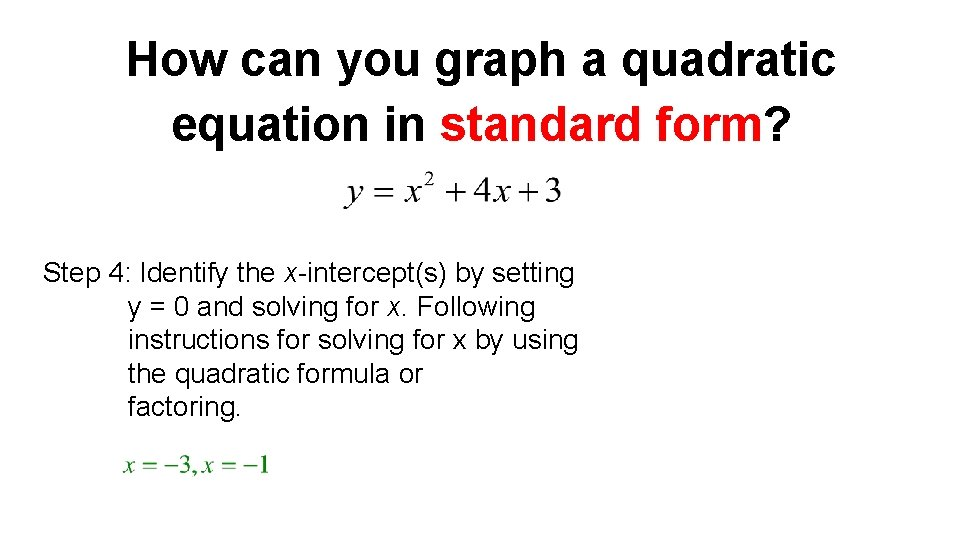How can you graph a quadratic equation in standard form? Step 4: Identify the x-intercept(s) by setting y = 0 and solving for x. Following instructions for solving for x by using the quadratic formula or factoring.How can you graph a quadratic equation in standard form? Step 5: Identify the y-intercept by setting x = 0 and solving for y. Step 6: Graph the intercepts.How can you graph a quadratic equation in standard form? axis of symmetry: vertex: x-intercepts: y-intercepts: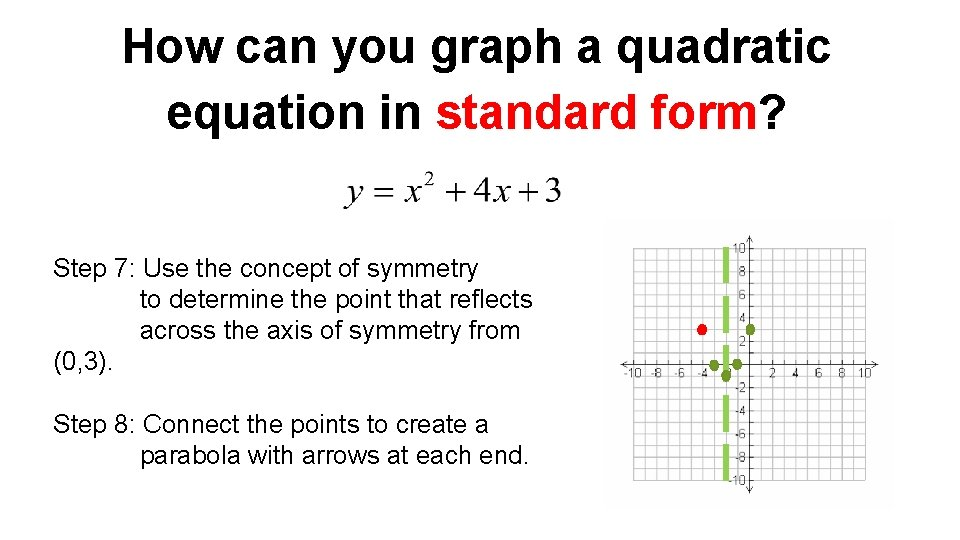How can you graph a quadratic equation in standard form? Step 7: Use the concept of symmetry to determine the point that reflects across the axis of symmetry from (0, 3). Step 8: Connect the points to create a parabola with arrows at each end.How can you graph a quadratic equation in standard form?What does a quadratic equation in vertex form look like?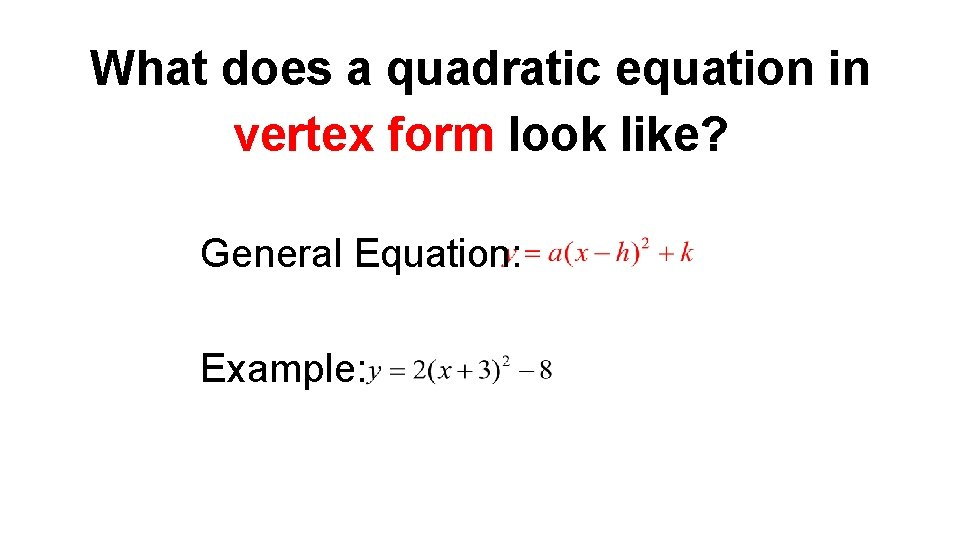What does a quadratic equation in vertex form look like? General Equation: Example:What are the advantages of a quadratic function in vertex form? • Vertex can easily be identified from the equation • Maximum and minimum can easily be identified from the equation • Easily shows how the graph is transformed fromHow can you graph a quadratic equation in vertex form? Step 1: Determine if the parabola opens up or down based on the value of a. Since a is negative, the parabola of this graph opens down. Step 2: The vertex of the graph can easily by determined by looking at the equation. Since x - h = x + 2, then h = -2. k = 4. The vertex of a parabola is (h, k), thus the vertex of thisHow can you graph a quadratic equation in vertex form? Step 3: Since we know the vertex lies on the axis of symmetry we can also determine the equation for the axis of symmetry. x = -2 Step 3: Watch a portion of the video (0: 00 – 2: 52) to learn how to use the a value to make a quick graph of a parabola. https: //www. youtube. com/watch? v=y 99 l. NRq. Lj. BA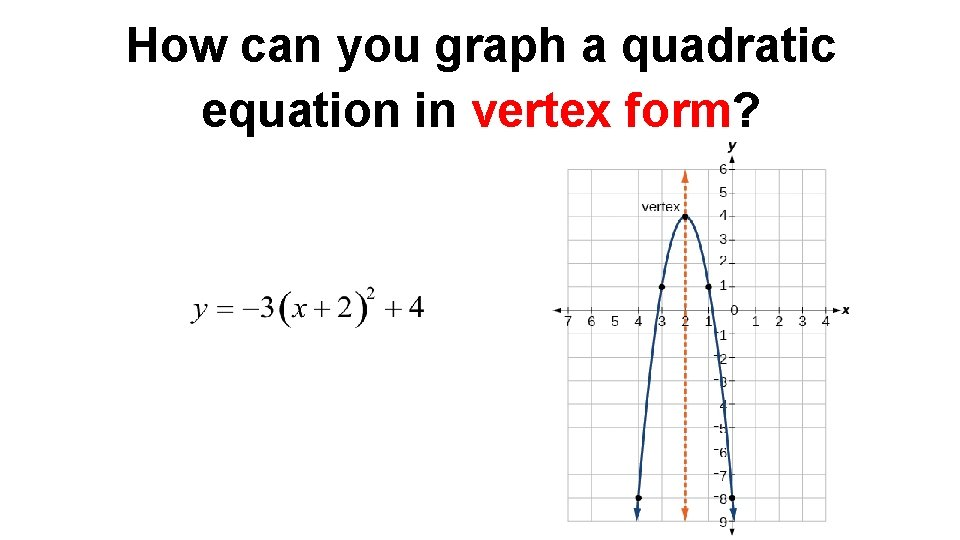How can you graph a quadratic equation in vertex form?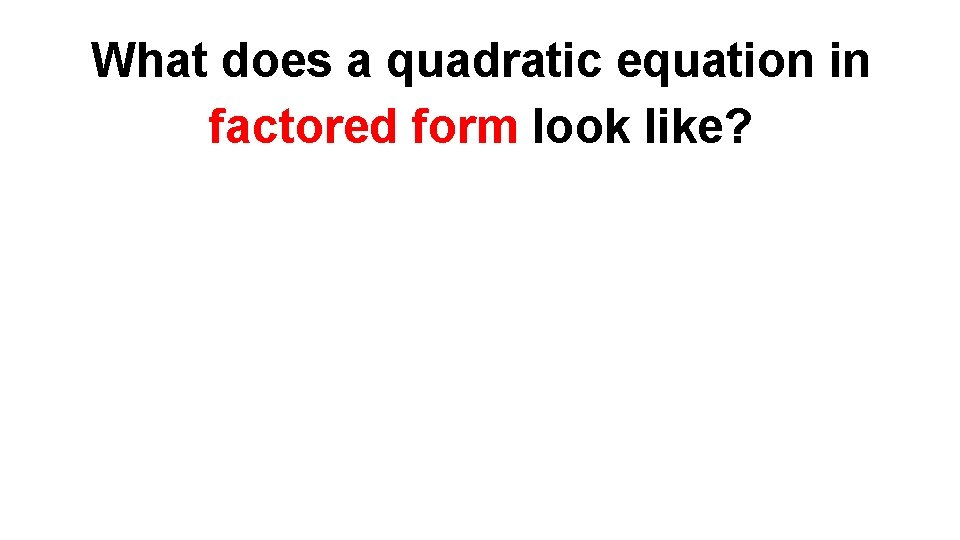What does a quadratic equation in factored form look like?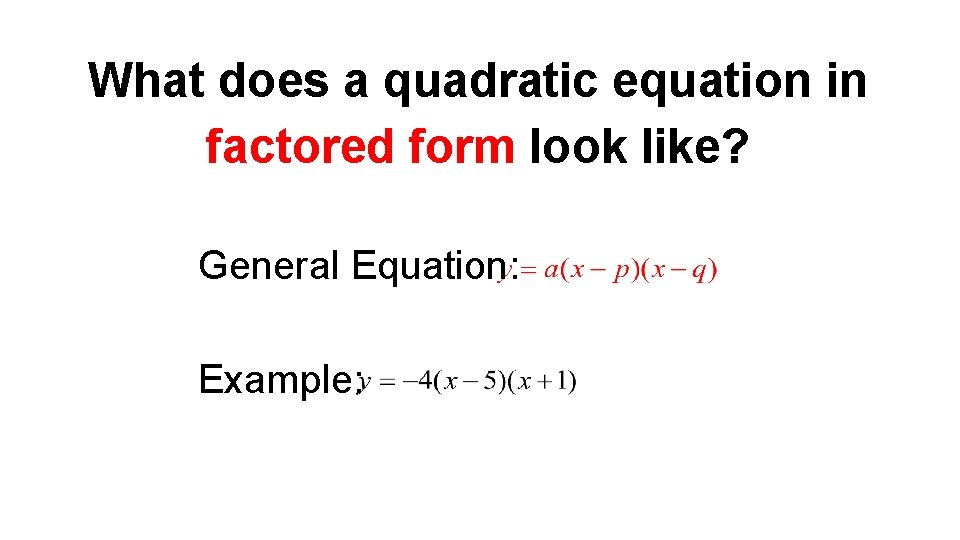What does a quadratic equation in factored form look like? General Equation: Example:What are the advantages of a quadratic function in vertex form? • Easily identifies the x-intercepts/roots/solutions of the quadratic function. • The axis of symmetry can easily be found using aHow can you graph a quadratic equation in vertex form? Step 1: Watch the entire video to see a quick example of graphing quadratic equations in vertex form. https: //www. youtube. com/watch? v=Ko. Qs. L 5 t_8 o 4 Step 2: Identify the x-intercepts/roots Step 3: Identify the equation of the axis of symmetry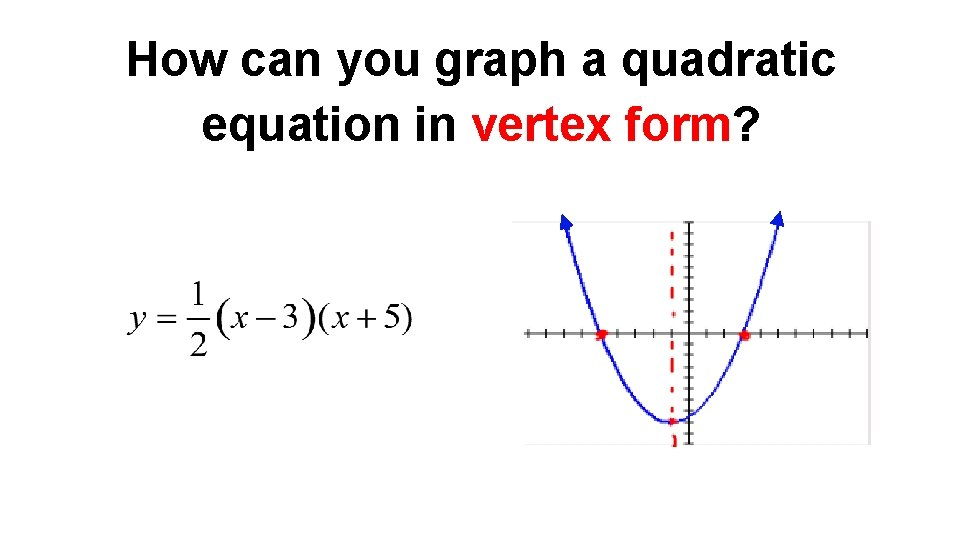How can you graph a quadratic equation in vertex form?INDEPENDENT PRACTICE EXAMPLE 1 Graph the equation below and include the key points.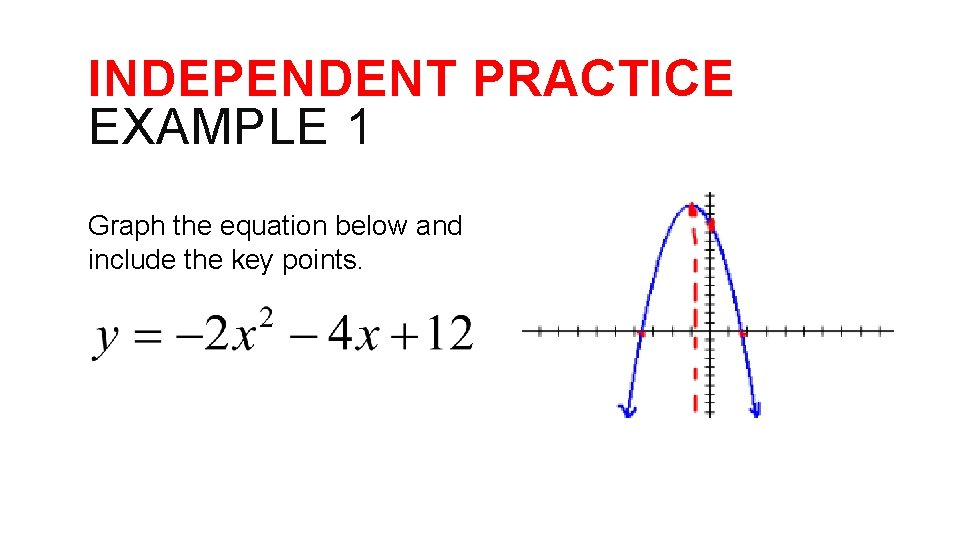INDEPENDENT PRACTICE EXAMPLE 1 Graph the equation below and include the key points.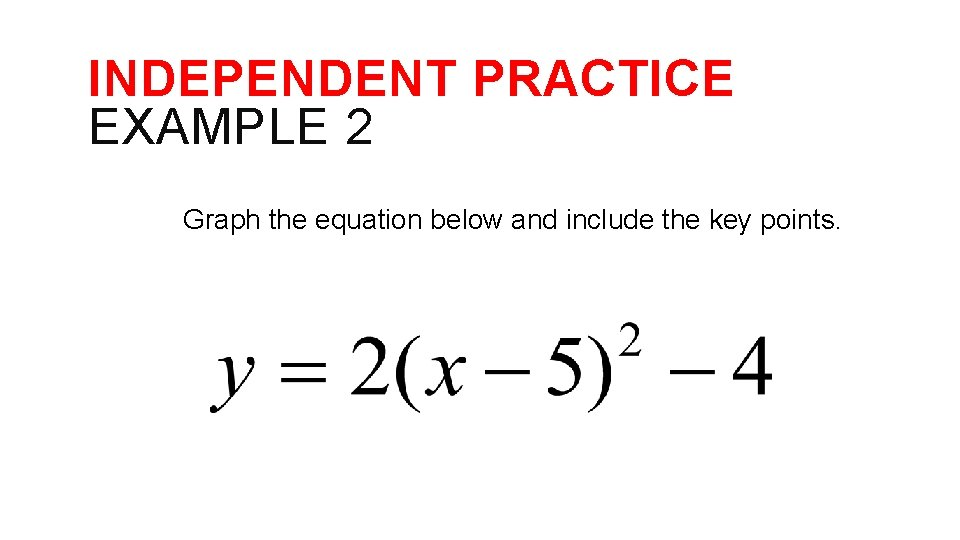INDEPENDENT PRACTICE EXAMPLE 2 Graph the equation below and include the key points.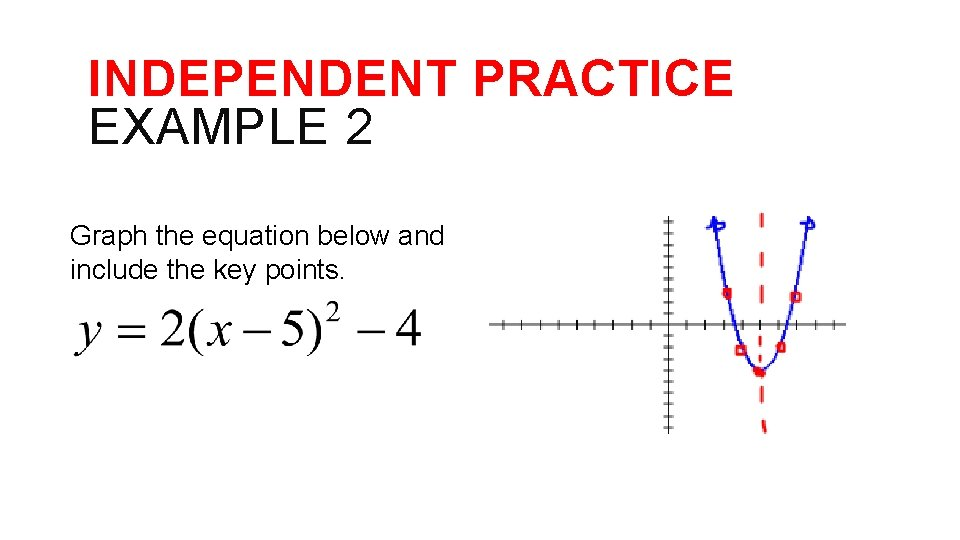INDEPENDENT PRACTICE EXAMPLE 2 Graph the equation below and include the key points.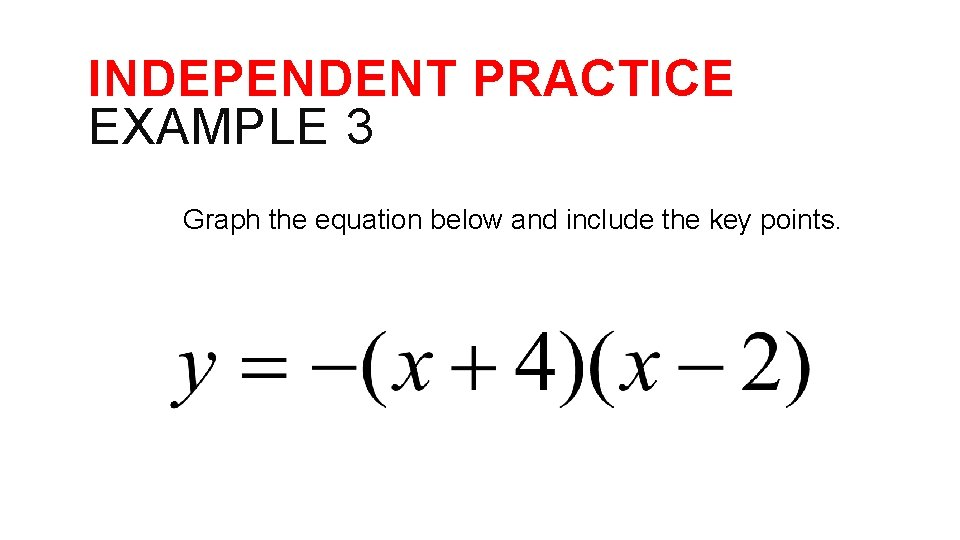INDEPENDENT PRACTICE EXAMPLE 3 Graph the equation below and include the key points.INDEPENDENT PRACTICE EXAMPLE 3 Graph the equation below and include the key points.